# Synchronous Rectification Circuit Section: Power Supply IC Selection

2019.12.25

・The operation and various conditions of the existing circuit are confirmed, and the power supply IC to be used in the design is selected.

・The maximum ON time is set to prevent failure due to simultaneous ON operation of MOSFETs on the primary and secondary sides.

・The compulsion OFF time is calculated, and the relevant BM1R001xxF series model is selected.

・In the BM1R001xxF series, five models with different compulsion OFF times are provided.

As part of a procedure to convert the secondary-side diode rectification circuit of the original circuit to synchronous rectification, in the previous article we selected a MOSFET to replace the rectifying diode. Following this, operation and various conditions of the preexisting circuit are verified, and the power supply IC to be used in the design is finalized. Here, design is being performed with the object of replacing the secondary-side diode rectification circuit of an existing AC-DC converter with a synchronous rectification circuit, and so an approach is necessary in which the specifications, characteristics and operation of the existing circuit are confirmed and adjustments are made, and components are then replaced to advance toward synchronous rectification.

## Synchronous Rectification Circuit Section: Power Supply IC Selection

The BM1R001xxF series that will be used in this replacement design consists of five models, the BM1R00146F through BM1R00150F. They offer a series of compulsion OFF times so as to enable synchronous rectification in power supplies with various specifications.

The compulsion OFF time prevents another turn-on operation of the secondary-side MOSFET due to the resonance waveform that occurs at the DRAIN pin of the IC under light loading. This time represents the interval, starting from when the secondary side turns off, during which turn-on is forcibly prevented; models with a compulsion OFF time of from 1.3 μs (BM1R00146F) to 4.6 μs (BM1R00150F) can be selected from the product lineup.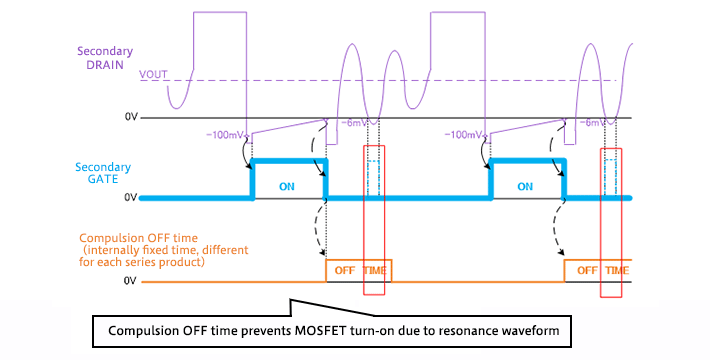However, if this compulsion OFF time is too long, there is a time interval during which the MOSFET cannot be turned on during heavy loading, so that efficiency is worsened. Hence an appropriate time must be selected (details will be explained later).

As criteria to be used when determining the compulsion OFF time during continuous mode operation, the switching frequency of the primary-side controller and the on-times of the MOSFETs on the primary side and secondary side must be considered.

The power supply IC is selected by following the procedures (1) to (3) below.

• (1)Check the waveforms of the reverse voltage VR and the forward current IF occurring in the rectifying diode DOUT prior to replacement
⇒Measure the ON-time t1 of the primary-side MOSFET M1 and one cycle tp of the primary-side controller
• (2)Set the maximum ON-time tMAX_ON of the secondary-side MOSFET
⇒The tMAX_ON setting prevents failure due to simultaneous on-operation of the primary-side and secondary-side MOSFETs under heavy loads while in continuous mode
• (3)IC selection
⇒Use the following equation to calculate the compulsion OFF time tOFF required, and select a model from the series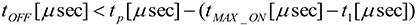This is a method for calculating the compulsion OFF time in continuous mode operation. A method of calculation for when continuous mode operation is not performed is described later. Please refer to this method as needed.

Below, details of each procedure are explained.

## (1) Check the waveforms of the reverse voltage VR and the forward current IF occurring in the rectifying diode DOUT prior to replacement

The waveform diagram on the right is an example of the waveforms of the reverse voltage VR and forward current IF occurring in a rectifying diode DOUT.

Conditions of the measurements are VIN＝400 Vdc and IOUT＝10 A (max).

From these waveforms, the following can be deduced.

• ・The ON-time of the primary-side MOSFET M1 is t1 = 1.4 μsec.
• ・One cycle of the primary-side controller is tp = 7.7 μsec.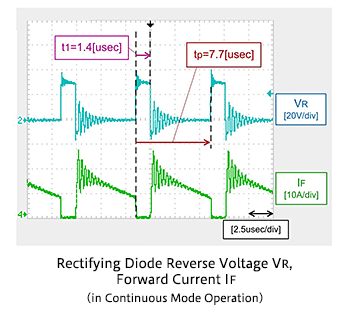## (2) Set the maximum on time tMAX_ON of the secondary-side MOSFET

The MAX_TON pin of the IC is used to set the maximum ON time tMAX_ON. When a rising voltage edge of at least VCC (= the output voltage VOUT) × 1.4 V typ is detected at the DRAIN pin, counting for the maximum ON time begins. When the maximum ON time tMAX_ON set using the resistor RTON has elapsed, the secondary-side MOSFET M2 is forcibly turned off.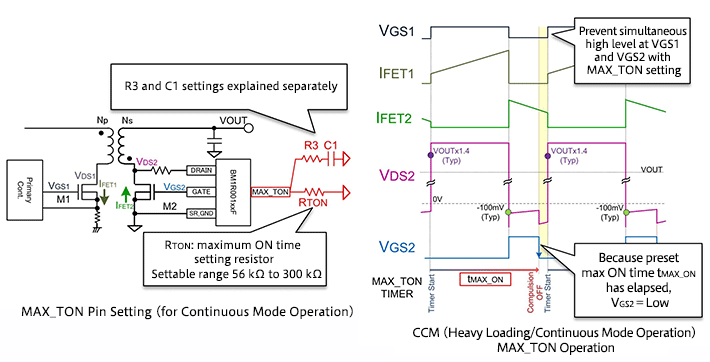The maximum ON time tMAX_ON must always be set to be shorter than one cycle tp of the primary-side controller, as shown in the figure below. The resistor RTON can be set in the range 56 kΩ to 300 kΩ, and tMAX_ON is proportional to this resistance value. The closer the set value of tMAX_ON to 10 μs (RTON=100 kΩ), the higher the resulting precision. Please refer to the graph below.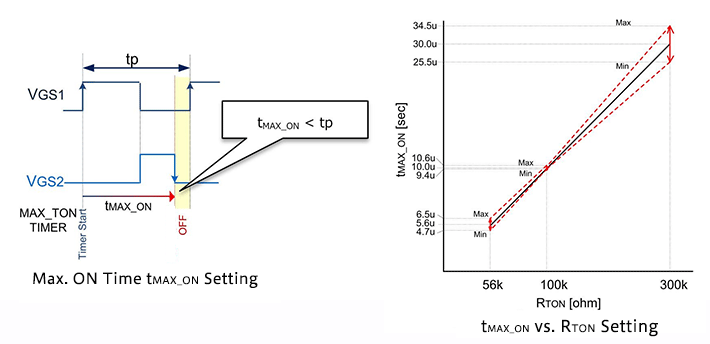If the primary-side controller uses PWM control, a value for RTON that takes variation into consideration is determined using the following equation.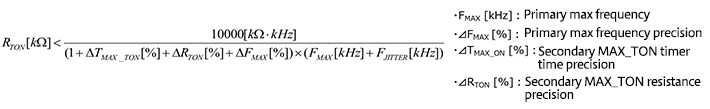In this design example, given FMAX＝130 kHz, ⊿FMAX＝5%, ⊿tMAX_ON＝7%, and ⊿RTON＝1%, RTON is as follows.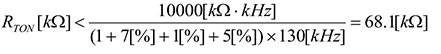In this design example, RTON is set to 68 kΩ or lower. However, this equation is for an ideal state, and thorough checking of the operation of actual equipment is necessary. If RTON is set to 68 kΩ, then tMAX_ON can be calculated using the following equation to be 6.8 μsec.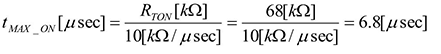## (3) IC selection

From the primary-side MOSFET M1 ON time t1 and the primary-side controller cycle time tp measured in (1), and from the maximum ON time tMAX_ON calculated in (2), the required compulsion OFF time tOFF can be calculated using the following formula.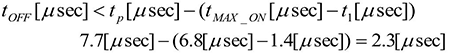Using the above calculation result and taking variation into consideration, the model BM1R00147F, with a compulsion OFF time tOFF of 2 μs (typ.), is selected. The table below shows compulsion OFF times tOFF for the BM1R001xxF series. Variation in compulsion OFF times is ±9%. Because this formula is for an ideal state, operation in actual equipment must be checked thoroughly before setting this value.

As an example, the continuous operation (under heavy loading) secondary-side synchronous rectification operation waveform using a BM1R00147F, and with RTON = 68 kΩ, is shown. It can be seen that after tMAX_ON has elapsed VGS2 turns off, and after tOFF it is again turned on.

Part # Compulsion OFF Time （Typ.）[μs]
BM1R00146F 1.3
BM1R00147F 2.0
BM1R00148F 3.0
BM1R00149F 3.6
BM1R00150F 4.6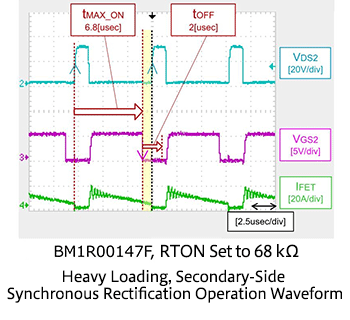Here, the optimum IC for conversion of an existing power supply to synchronous rectification can be selected. This example assumes continuous mode operation, but for reference we include considerations for a case in which the existing power supply does not operate in continuous mode and a case in which the primary-side controller is provided with a jitter function.

## * Method of calculating the compulsion OFF time when operation is not in continuous mode and MAX_TON pin settings

In a discontinuous mode and under quasi-resonant control, continuous-mode operation does not occur, and so MAX_TON pin settings are unnecessary. Hence the maximum ON time tMAX_ON is not set, and so there is no need to calculate tMAX_ON. Instead, the time tON from the time the rectifying diode turns on under maximum loading until the forward current IF reaches zero is measured. The required compulsion OFF time is found from the following formula.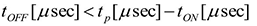A waveform example is shown. In this case the MAX_TON pin is directly pulled up to VCC.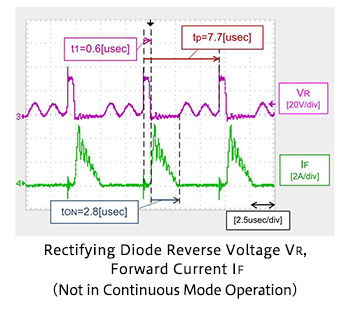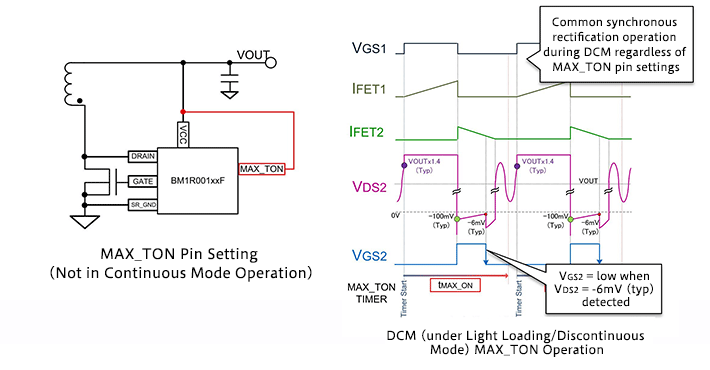When in discontinuous mode, synchronous rectification operation is the same regardless of MAX_TON pin settings. Further, when VDS2 reaches -6 mV (typ.), VGS2 turns off.

## * Case in which the primary-side controller is provided with a jitter function

The setting of RTON taking variation into account in a case in which the primary-side controller is provided with a jitter function is based on the following equation.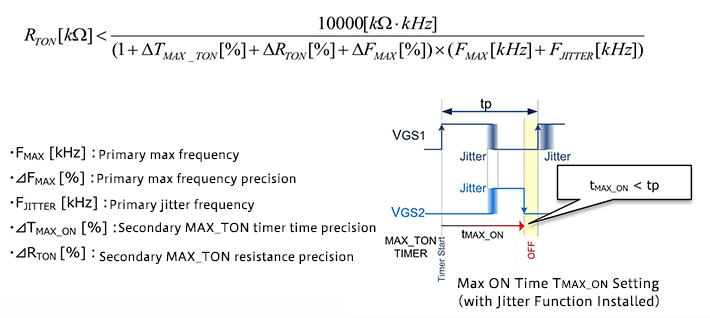In the continuous-mode equation presented above, a primary-side jitter frequency FJITTER (kHz) is added to the denominator.